# Inverting amplifier

The inverting opamp inverts the input terminals, so the input voltage is connected to the negative terminal and the positive terminal is connected to ground. If the input voltage is positive, the output voltage will be negative.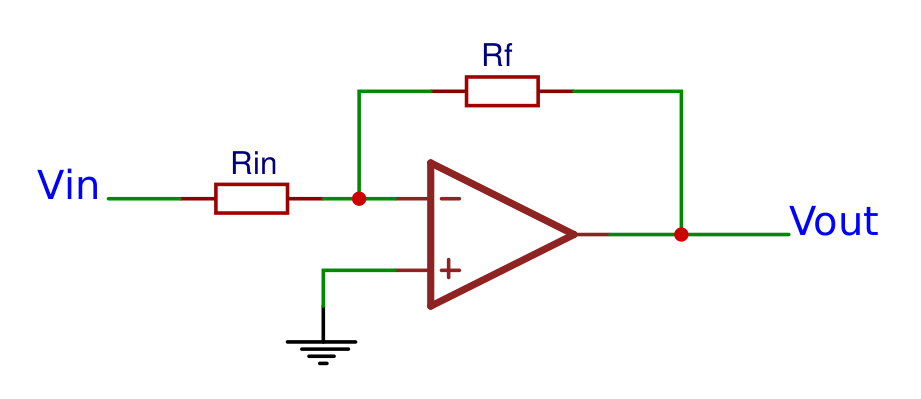### Formulas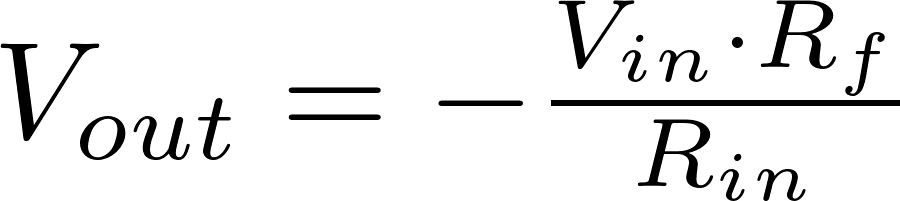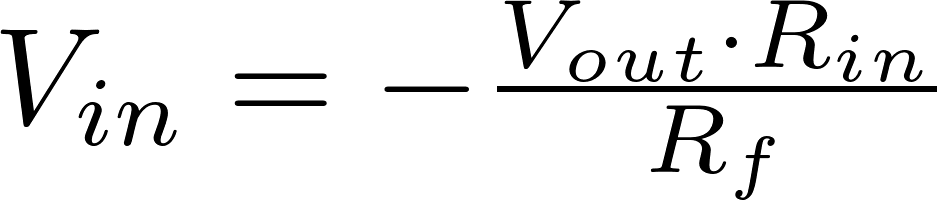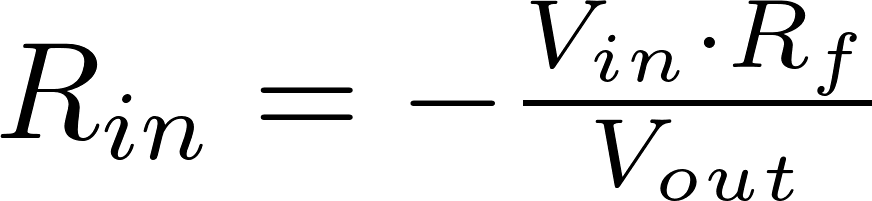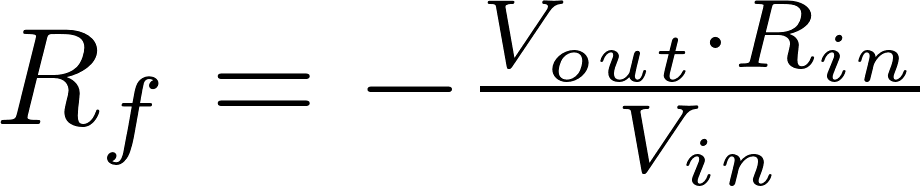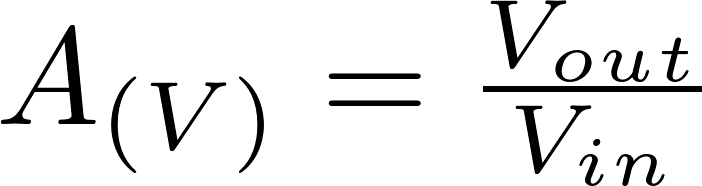V in is the input voltage and is measured in volt (V)
V out is the output voltage and is measured in volt (V)
R is the symbol for resistance and is measured in ohm (Ω).
A is the symbol for gain.